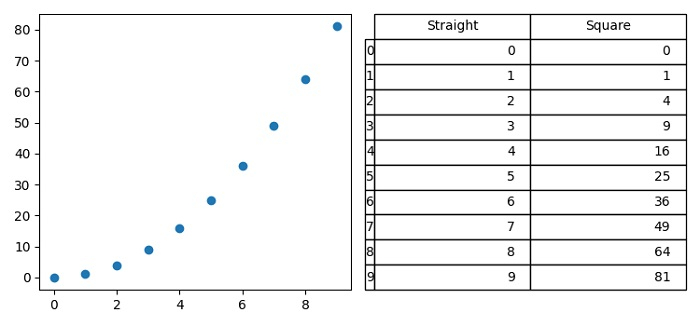# How to display a Dataframe next to a Plot in Jupyter Notebook?

To display a dataframe next to a plot, we can take the following steps −

• Set the figure size and adjust the padding between and around the subplots.

• Make a Pandas dataframe with straight and square keys.

• Create a new figure or activate an existing figure using figure() method.

• Add a subplot to the figure with nrows=1, cols=2 and index=1.

• Plot dataframe points using scatter() method.

• Add subplot to the figure with nrows=1, cols=2 and index=2.

• Initialize variables font_size, bbox to make a table.

• Turn off the current axes.

• Add a table to the current axis using table() method.

• To display the figure, use show() method.

## Example

import matplotlib.pyplot as plt
import pandas as pd

plt.rcParams["figure.figsize"] = [7.50, 3.50]
plt.rcParams["figure.autolayout"] = True

df = pd.DataFrame({'Straight': [i for i in range(10)],'Square': [i * i for i in range(10)]})

fig = plt.figure()
plt.show()